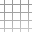#Jan Varho

## Fast Approximate Move-to-Front

• algorithm
• coding
• MTF
• pypy
• Python

Move-to-front is an algorithm for coding symbols that have been seen recently with a smaller number. It’s used as a part of some data compression algorithms, e.g. after a Burrows-Wheeler transform.

I tried to approximate it in a faster way and found an algorithm that I haven’t seen elsewhere (although it’s probably not new).

### Move-to-Front

In move-to-front, the encoder and decoder maintain a list of symbols, from most to least recently seen. When a new symbol is seen, that symbol is moved to the top of the list, and gets the index 0 or 1, which if seen again can be encoded with few bits, e.g. using delta coding.

The simplest implementation is an array, but in the worst case you have to move all the elements of the array, to get to least recently seen element to the top of the list. A linked list is just as bad, because to find a symbol you may have to traverse the whole list. A tree can give you logarithmic performance, but at the expense of complexity and memory use.

Here’s a simple array implementation of the encoder in Python:

def mtf_put(s): global mtf_ind, mtf_sym

i = mtf_ind[s]

for j in range(i): tmp = mtf_sym[i-j] = mtf_sym[i-j-1] mtf_ind[tmp] += 1

mtf_sym = s mtf_ind[s] = 0

return i

If the alphabet is small, e.g. bytes, and most of the indices are small, performance of the array implementation is in practice quite good. However, I wanted to apply it to a large variable length alphabet, and would like constant performance. That’s where approximations come into play.

### Approximate Move-to-Front

Most of the time in the array implementation is spent on moving symbols other than the one encountered to their correct places. The simplest way to avoid that would be to simply swap the symbol with the top one, but that obviously won’t work – the array will not sort, only the most recently used symbol is guaranteed to be at the top of the list, the rest can be anywhere.

A better try is to make the list cyclic. Put the newly seen symbol in front of the previous list head and update a head pointer. To avoid unbounded list growth, you can take the last symbol of the list and place it where the current symbol used to be. That introduces some "random" noise, but still lets the list sort partially:

def amtf_put(s): global N, mtf_ind, mtf_sym, mtf_pos

i = mtf_ind[s] n = (mtf_pos - i) % N

mtf_pos = (mtf_pos + 1) % N tmp = mtf_sym[i] = mtf_sym[mtf_pos] mtf_ind[tmp] = i

mtf_sym[mtf_pos] = s mtf_ind[s] = mtf_pos

return n

How good is this approximation? Intuitively, every time a symbol is moved to front, another symbol is also moved somewhere close to the front, so the algorithm is "half as good". With the correct symbol distribution that is indeed the case and the average index value about doubled (see below).

As a guarantee, a symbol that’s been seen within the previous X symbols is guaranteed to be within the first X symbols in the list, so it is at least as good as encoding backreferences.

### Better Approximation?

Is there a way to improve it? A small optimization is to leave the list untouched when you see the most recent symbol again, but that only affects things if runs are very common (like after BWT).

A more effective change is to make another move when updating the array. Instead of moving the last symbol to the old position of the current one, move some other symbol from index M to that position, then move the last to position M. However, this should only be done when the current symbol was ahead of the index M, otherwise the list from M to the end is just being cycled with no order:

def amtf_put(s): global N, M, mtf_ind, mtf_sym, mtf_pos

i = mtf_ind[s] n = (mtf_pos - i) % N

if n == 0: return 0 elif n < M: mpos = (mtf_pos - M) % N mtf_pos = (mtf_pos + 1) % N tmp = mtf_sym[i] = mtf_sym[mpos] mtf_ind[tmp] = i tmp = mtf_sym[mpos] = mtf_sym[mtf_pos] mtf_ind[tmp] = mpos else: mtf_pos = (mtf_pos + 1) % N tmp = mtf_sym[i] = mtf_sym[mtf_pos] mtf_ind[tmp] = i

mtf_sym[mtf_pos] = s mtf_ind[s] = mtf_pos

return n

Which position M you should choose depends, again, on the symbol distribution. (TODO: Calculate the optimal M for when the symbol distribution is e.g. truncated exponential.)

The same guarantee as above applies – a symbol seen within X symbols is within the first X indices, because symbols can only move back by one slot.

### Some Data

Trying to encode enwik8 using only bytewise MTF results in a mean index of 15.1. The 1-move approximation has a mean index of 34.1, or 33.1 when keeping the list constant with repeated symbols. The two move approximation has a minimal mean of 22.1 with M = 68 and values of M in the range [63,73] all have mean less than 22.2.

Calculating the median index instead, for MTF the result is 10, for 1-move AMTF 14, and for 2-move AMTF a minimum of 11 (for M in the range [9,20]).

Running times with pypy go from 10-11 seconds for MTF to 1-2 seconds for either AMTF. CPython is orders of magnitude slower on this kind of code, 287-288 seconds for MTF, 54-55 seconds for 1-move AMTF and 69-70 seconds for 2-move AMTF.